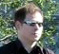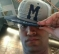# Polyphase filter / Farrows interpolation

Hello,

A good reference with more depth is for example Fred Harris' paper: http://www.signumconcepts.com/IP_center/paper018.pdf

Interpolate a band-limited discrete-time signal at a variable offset between samples.
In other words:
Delay the signal by a given amount with sub-sample accuracy.
Both mean the same.

The picture below shows samples (black) representing a continuous-time waveform (blue).Conceptually, the problem is "easy" to solve:

Reconstruct the continuous-time waveform from the samples,
then
resample as desired

Ideal reconstruction of the blue curve requires an ideal lowpass filter. It can be approximated by a FIR filter to any desired accuracy (but the better the filter, the more latency is introduced to the system).

Another approximation can be made: Instead of delaying the signal in arbitrary small steps, it may be sufficient to limit the accuracy to a fraction 1/N of a sample. In my example I'm using 1/8.
This limitation will be lifted at the end, when the polyphase filter is developed into the Farrows interpolator.

Interpolate the signal by a factor of N (in the example 8)
and
decimate with an offset according to the delay, also called "decimation phase".

Interpolation is done by inserting zero samples... (picture)... and lowpass-filtering (picture).Out of N samples, the one with the desired offset is now picked out. The other N-1 samples are discarded by decimation.

For example: decimation for a 1/8 offset, only the red samples 'survive':Alternative decimation phase: Delay 2/8 samples, the green samples are of interest:Another decimation phase for 3/8 samples delay (blue samples)A possible FIR filter implementing the lowpass and decimation is shown below:
For the 1/8 delay case, only the red taps are nonzero, the other samples are discarded (decimated):
Delay by 2/8 uses only the green taps, delay by 3/8 only the blue taps and so on.A real FIR filter designed to typical requirements (most notably passband ripple, alias rejection, width of the transition region), may easily require 100 taps or more. It will be much longer than shown.

Of course, an implementation according to above picture would be very inefficient, since most parts of the filter aren't doing anything (not contributing to the output).
This can be seen in the following picture, showing the same filter with all "decimated" branches removed.Only one out of 8 taps is nonzero. Also, N-1 zero samples are created at the input for every actual sample, and they cannot contribute to the output.
As a result, the filter can be simplified, and the N-1 zero samples per input sample are omitted.
The picture below shows three filters for different decimation phase, delaying the signal by 1/8, 2/8 or 3/8 of a sample, respectively.Note that in comparison to the full-length FIR filter, the length has shrunk by a factor of N (8 in the example). Only one of the above filters is needed at a time. So one filter is sufficient, with N banks of coefficients that are selected by a "commutator switch".Even though the number of delays and multipliers has been reduced, the total number of coefficients remains the same as in the original filter.

The higher the required subsample accuracy N, the more coefficients are needed.
Instead of stacking more and more coefficients for each multiplier, it is possible to fit one polynomial to the series of coefficients for each multiplier. Its variable is the desired subsample delay, corresponding to the position of the commutator switch in the above picture.

The Farrows interpolator replaces each column of coefficients with a polynomial.

So much for now...
I hope the material has been useful to give some overview on the topic.
Especially "bug reports" are always welcome.

Cheers

Markus

Another free reference: http://www.acoustics.hut.fi/~vpv/publications/icassp00-fd.pdf

PS: Be careful with using equiripple filter designs (Parks McClellan) for Farrows scheme.
The outermost points of the impulse response do not form a "smooth" curve. They are a discontinuity and cannot be easily approximated by a polynomial.
This issue does not appear with a simple polyphase implementation of the same filter.

Some related code snippets:

Determining the delay between two given signals and resampling

Farrow resampler implementation in plain Matlab (vectorized, no toolboxes)

Farrow resampler implementation in C (sample-by-sample)

Ideal resampling at arbitrary time instants

Bank-switched Farrow resampler: (documented here): Fewer multiplications at the expense of more coefficients

[ - ]
Comment by October 2, 2007hello! Do you know linear interpolation of linear prediction coefficients in speech coding?. If you know that, could you explain it to me? Thanks a lot
[ - ]
Comment by October 2, 2007Hello, sorry, my knowledge in that area is quite basic. But maybe one of the other bloggers would like to pick up the topic?
[ - ]
Comment by October 3, 2007Markus, I'm looking forward the the Farrows Interpolation details that I assume will follow. How doe this technique compare with Polyphase IIR methods implemented using allpass subfilters - described in "DSP System Design" by Krukowski and Kale ?
[ - ]
Comment by October 8, 2007Hello, a good place for details regarding Farrows interpolation is the reference at the beginning of the article. I think I won't go any deeper into the details here. About FIR/IIR, it's a tricky question. Which one is better? Maybe it's best to compare alternative designs for a particular set of requirements. In general, FIRs have the advantage of linear phase, but IIR allpasses can reportedly achieve "almost" linear phase. The advantage of IIR filters is almost instantaneous response, whereas FIR filters pay for the linear phase with longer latency. Maybe I should add one comment: If I were faced with a problem that requires a Farrows interpolator, the first thing I'd do is to have a look at the system design and try to get rid of it...
[ - ]
Comment by March 23, 2021The reference cannot be reached. Can you present the full title of the paper so that I can download it from the Internet? Thanks in advance

[ - ]
Comment by October 10, 2007Great article!! Please keep up the good work
[ - ]
Comment by October 16, 2007How are these coefficients generated ?Can anyone give some pointers to this? Is there any way to use the same coefficients to use for all the other delay paths and move them circularly for the result to be delayed more or less ?
[ - ]
Comment by October 21, 2007Hello, the coefficients are obtained with standard FIR filter design methods, for example the filter design tool in Matlab. There is the usual tradeoff between passband ripple, stopband attenuation and filter size. And yes, a polyphase filter can be used as a variable delay line. For example, the red, green and blue coefficient sets would correspond to three different delays. Use the output samples that correspond to one bank (for example "green") and simply don't calculate the others (red, blue, ...).
[ - ]
Comment by September 15, 2008HI Markus, As you said that the coefficients {c0,c1,c2.....cN}are obtain with standard FIR filter design method. But what's means that "The Farrows interpolator replaces each column of coefficients with a polynomial"? Following is my understanding, please help me confirm. For get more accurate interpolated result, we need a polynomial interpolation based on the different filter bank result? Follow your example, if we need to get the value at (1/8+u) where 0
[ - ]
Comment by September 15, 2008Sorry, why I can not paste all my comment one time. Follow your example, if we need to get the value at (1/8+u*1/8). where u is a fraction number between 0 and 1. so we need apply a polynomial interpolator on the different filter bank's result. For exmaple, linear interpolation, result = red_result*u+green_result*(1-u) . is my understanding right?
[ - ]
Comment by September 17, 2008Hello, yes, that's how it works. The "stack" of red/green/blue coefficients doesn't have the desired resolution, therefore I interpolate between them. This is from the Fred Harris reference, page 2: "A third option is to approximate the filter weights in a segment of its impulse response by a low order polynomial and then use the polynomial to compute the filter weights at their desired positions"
[ - ]
Comment by June 28, 2010Hi Markus nice discussion. I would like to give me more light on this subject, I need to approximate continuous time signal from original discrete samples using polynomial-based interpolation(Farrow structure) filter, my problem is on the branch filters.suppose in my analysis I plan to use order 4 polynomial interpolation, hence I would need 5 FIR branch filters, are these branch filters designed with similar specification or?besides we can simply perform interpolation with one FIR filter example square root raised cosine filter,so why do we need polyphase filter? this is very important as I need to use farrow filter in timing synchronization I appreciate your help inadvance!
[ - ]
Comment by July 23, 2014Hello,

this technique is valid for interpolation, but for the decimation as you progress ?

Is possible to have the code "C" for decimation ?

debugasm
[ - ]
Comment by July 23, 2014Hi,

looking at the math, interpolation and decimation are the same: "m" input samples generate "n" output samples, the conversion ratio is n/m regardless of which one is greater.
A useful generic C implementation is somewhat difficult as it depends heavily on its surroundings (i.e. block processing).
For example, you can find example "C" code (a 256-bank polyphase using Catmull-Rom splines) in the open source project "fluidsynth" in the dsp/voice routines. For audio, this can work fairly well.

I've got some _RTL_ code for a polyphase resampler that does both interpolation and decimation, here:
http://www.dsprelated.com/showarticle/198.php

To post reply to a comment, click on the 'reply' button attached to each comment. To post a new comment (not a reply to a comment) check out the 'Write a Comment' tab at the top of the comments.